1. Aluminum metal reacts with zinc chloride to produce zinc metal and aluminum chloride.

Balance the equation.

2Al + 3ZnCl2 → 3Zn + 2AlCl3

a. A mass of 45.0 grams of aluminum will react with how many grams of zinc chloride?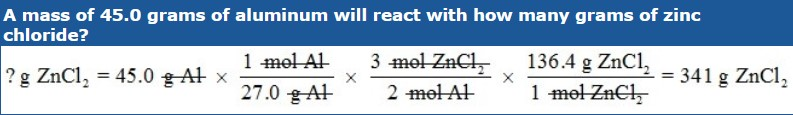b. What mass of aluminum chloride will be produced if 22.6 grams of zinc chloride are used up in the reaction?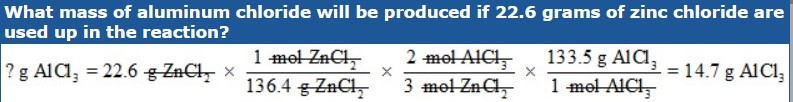For the reaction whose balanced equation is as follows, find the number of grams of I2 that will be formed when 300.0 g of bromine react.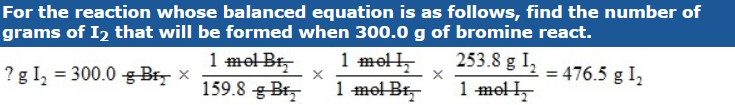For the reaction whose balanced equation is as follows, find the number of grams of sodium that must react to produce 42.0 grams of sodium oxide.For the reaction whose balanced equation is as follows, find how many grams of zinc phosphate will be produced by the reaction of 5.00 grams of ammonium phosphate.When mercury (II) oxide is heated, it decomposes into mercury and oxygen gas according to the following BALANCED equation.

2 HgO → 2 Hg + O2

a. Given that the density of oxygen is 1.439 g/L, how many liters of oxygen gas can be produced if 2.0 moles of mercury (II) oxide are heated?b. How many molecules of oxygen gas are produced if 25.0 g of mercury (II) oxide are heated?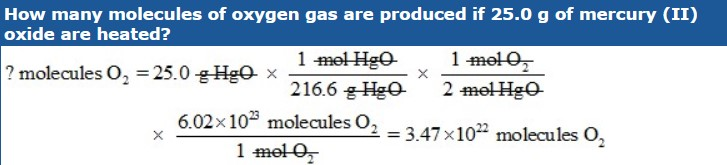How many molecules of sodium nitrate are produced when 20.0 g of sodium azide, NaN3, react according to the following BALANCED equation?

NaN3 + AgNO3 → AgN3 + NaNO3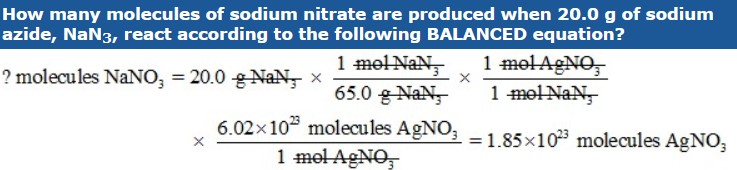Chlorobenzene, C6H5Cl, is used in the production of chemicals such as aspirin and dyes. One way that chlorobenzene is prepared is by reacting benzene, C6H6, with chlorine gas according to the following BALANCED equation.

C6H6 (l) + Cl2 (g) → C6H5Cl (s) + HCl (g)

a. What is the theoretical yield if 45.6 g of benzene react?b. If the actual yield is 63.7 g of chlorobenzene, calculate the percent yield.When carbon disulfide burns in the presence of oxygen, sulfur dioxide and carbon dioxide are produced according to the following equation.

CS2 (l) + 3 O2 (g) → CO2 (g) + 2 SO2 (g)

a. What is the percent yield of sulfur dioxide if the burning of 25.0 g of carbon disulfide produces 40.5 g of sulfur dioxide?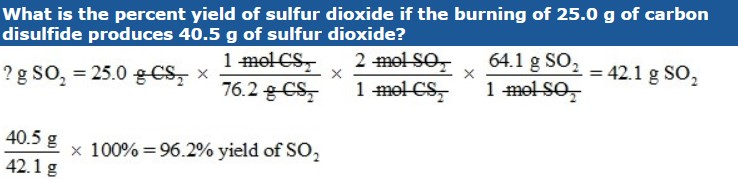b. What is the percent yield of carbon dioxide if 2.5 mol of oxygen react and 32.4 g of carbon dioxide are produced?BALANCE the equation first.

FeCl3 + O2 → Fe2O3 + Cl2

a. How many moles of chlorine gas can be produced if 4 moles of FeCl3 react with 4 moles of O2?

? mol Cl2 = 4 mol FeCl3 (6 mol Cl2 / 4 mol FeCl3) = 6 mol Cl2

? mol Cl2 = 4 mol O2 (6 mol Cl2 / 3 mol O2) = 8 mol Cl2

b. What is the limiting reactant?
FeCl3

c. What is the excess reactant?
O2

Use the following BALANCED equation.

2 C2H6 + 7 O2 → 4 CO2 + 6 H2O

a. If 15 g of C2H6 react with 45 g of O2, how many grams of water will be produced?

? g H2O = 15 g C2H6 (1 mol C2H6 / 30.0 g C2H6) x (6 mol H2O / 2 mol C2H6) x (18.0 g H2O / 1 mol H2O) = 27 g H2O

b. What is the limiting reactant?

O2

c. What is the excess reactant?
C2H6

source